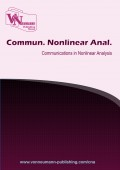# Solutions for some nonlinear functional-integral equations with applications

Volume 5, Issue 1, pp 28--39 Publication Date: 2018-08-14### Authors

Animesh Gupta - Department of Mathematics & Computer Science, R.D.V.V. Jabalpur (M.P.) India.
Saurabh Manro - Department of Mathematics, Thapar University, Patiala, Punjab, India.
Rashmi Rani - Department of Mathematics, A.S. College for Women, Khanna, Punjab, India.

### Abstract

In the present manuscript, we prove some results concerning the existence of solutions for some nonlinear functional-integral equations which contains various integral and functional equations that considered in nonlinear analysis and its applications. By utilizing the techniques of noncompactness measures, we operate the fixed point theorems such as Darbo's theorem in Banach algebra concerning the estimate on the solutions. The results obtained in this paper extend and improve essentially some known results in the recent literature. We also provide an example of nonlinear functional-integral equation to show the ability of our main result.

### Keywords

Banach algebra, fixed point, functional-integral equation, measure of noncompactness

### References

•  J. Bana´s, K. Goebel, Measures of Noncompactness in Banach Spaces, Lecture Notes in Pure and Applied Mathematics, Marcel Dekker, New York (1980)

•  J. Bana´s, M. Lecko, , Fixed points of the product of operators in Banach algebra, Panamer. Math. J., 12 (2002), 101-109

•  J. Bana´s, M. Mursaleen, Sequence spaces and measures of noncompactness with applications to differential and integral equations, Springer, New York (1981)

•  J. Bana´s, B. Rzepka, An application of a measure of noncompactness in the study of asymptotic stabilit, Appl. Math. Lett., 16 (2003), 1-6

•  J. Bana´s, B. Rzepka, On existence and asymptotic stability of solutions of a nonlinear integral equation, J. Math. Anal. Appl., 284 (2003), 165-173

•  J. Bana´s, B. Rzepka, On local attractivity and asymptotic stability of solutions of a quadratic Volterra integral equation, Appl. Math. Comput., 213 (2009), Appl. Math. Comput., 213 (2009), 102-111

•  J. Bana´s, K. Sadarangani, Solutions of some functional–integral equations in Banach algebra, Math. Comput. Modelling, 38 (2003), 245-250

•  S. Chandrasekha, Radiative Transfer, London, Oxford Univ Press (1950)

•  B. C. Dhage, On α–condensing mappings in Banach algebras, Math. Student, 63 (1994), 146-152

•  D. Guo, V. Lakshmikantham, X. Z. Liu, Nonlinear Integral Equations in Abstract Spaces, Kluwer, Dordrecht (1996)

•  ] S. Hu, M. Khavani, Integral equations arising in the kinetic theory of gases, Appl. Anal., 34 (1989), 261-266

•  K. Maleknejad, R. Mollapourasl, Study on existence of solutions for some nonlinear functional integral equations, Nonlinear Anal., 69(8) (2008), 2582-2588

•  K. Maleknejad, K. Nouri, R. Mollapourasl, Existence of solutions for some nonlinear integral equations, , Commun. Nonlinear Sci. Numer. Simul., 14 (2009), 2559-2564

•  K. Maleknejad, K. Nouri, R. Mollapourasl, Investigation on the existence of solutions for some nonlinear functional- integral equations, Nonlinear Anal., 71 (2009), el575-el578

•  J. J. Nieto, R. Rodriguez-Lopez, Contractive mapping theorems in partially ordered sets and applications to ordinary differential equations, Order, 22 (2005), 223-

•  J. J. Nieto, R. Rodriguez-Lopez, Existence and uniqueness of fixed point in partially ordered sets and applications to ordinary differential equations, Acta Math. Sin.(Engl. Ser.), 23 (2007), 2205-2212

•  A. C. M. Ran, M. C. B. Reurings, A fixed point theorem in partially ordered sets and some applications to matrix equations, Proc. Amer. Math. Soc., 132 (2004), 1435-1443

•  B. Samet, C. Vetro, Coupled fixed point theorems for multi-valued nonlinear contraction mappings in partially ordered metric spaces, \ Nonlinear Anal., 74 (2011), 4260-4268